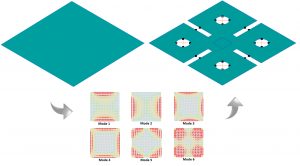Starting from the MoM impedance matrix for a given metallic structure, Characteristic Mode Analysis (CMA) solves an eigenvalue equation providing a weighted set of orthogonal current modes supported on the particular structure. The modes depend only on the physical parameters of the structure, not on the way the structure is excited.

In WIPL‑D only the boundary surfaces of a structure are meshed, mesh elements are quadrilateral, and Higher Order Basis Functions (HOBFs) are used to approximate the unknown current distribution. This set of features leads to significant reduction of the number of unknowns in the eigenvalue equation compared with some other numerical methods. Reduced number of unknowns results in very fast MoM matrix calculation and makes the particular CMA Solver implemented in WIPL‑D software highly efficient.When solving the eigenvalue equation, eigenvalues and a number of associated eigenvectors are calculated. Starting from eigenvalues, the modal significance and characteristic angle are calculated, two very important parameters providing an insight into physical behavior of the observed modes. Characteristic mode currents, and in turn related radiation patterns and near field distributions produced by the currents, are calculated from the eigenvectors.

In order to ensure that the modes with the same index number at different frequencies refer to the same physical modes, an advanced mode tracking technique is implemented into WIPL‐D CMA solver.

Visualization of the characteristic mode currents, radiation patterns and near fields can significantly increase the understanding of the physical behavior of the analyzed structure. For an example, driven by the graphic representation of calculated eigenvalue spectrum and the eigenvector distribution, a designer can intuitively get an idea how to bring the simulated structure to desired performance. Therefore, the CMA solver opens the possibility for a competent engineer to take the shortcut towards meeting the design goals, rather than relying on inefficient cut and try approach or a blind operation of an optimizer.